# 集中阻尼弦本征解的性质 *

(重庆交通大学 省部共建山区桥梁及隧道工程国家重点实验室， 重庆 400074)

## 1 集中阻尼弦的本征解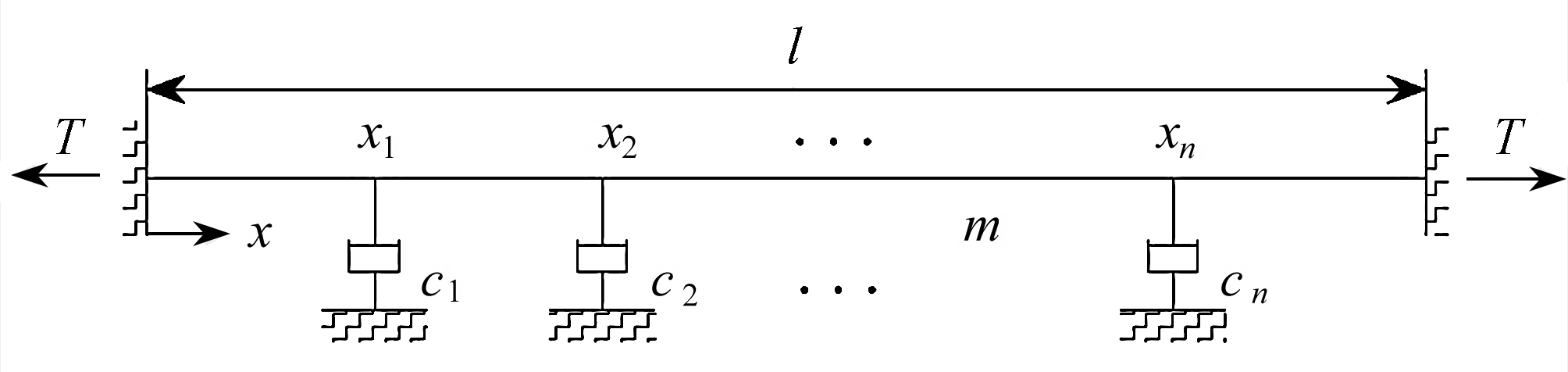Fig. 1 A taut string with n concentrated viscous damping dashpots

### 1.1 动力学平衡方程与分离变量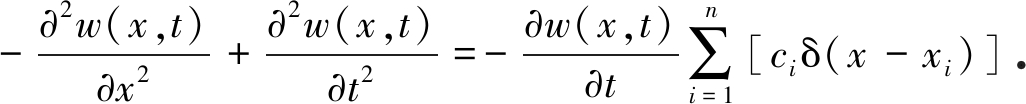(1)

φ(x)为w(x,t)的本征函数，采用分离变量法，由式(1)可得如下的常微分方程：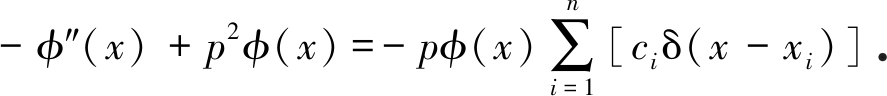(2)

### 1.2 加权和函数形式的本征函数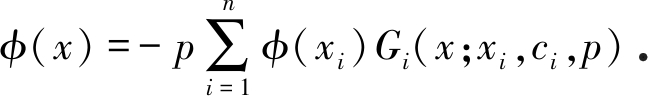(3)

-Gicp(x)+p2Gicp(x)=ciδ(x-xi)

(4)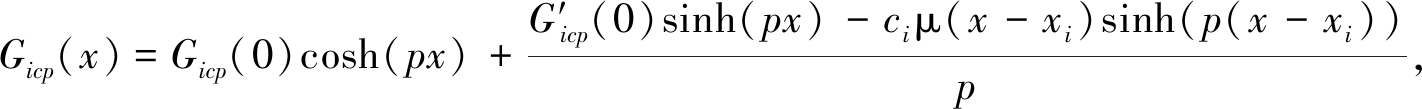(5)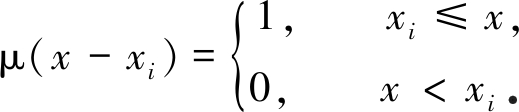(6)

### 1.3 位移协调条件、本征方程组与频率方程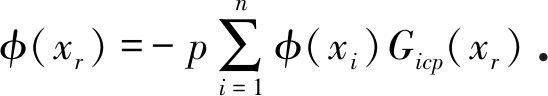(7)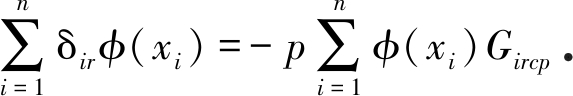(8)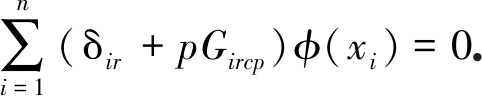(9)

(I+H)φ=0．

(10)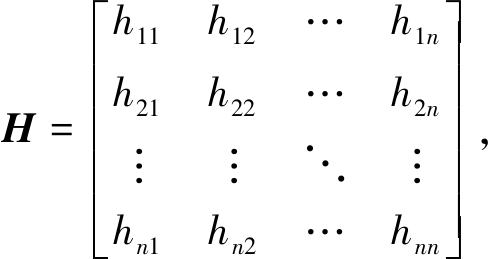(11a)

hir=pGircp

(11b)

det(I+H)=0

(12)

## 2 单集中阻尼弦的本征解

-φ″(x)+p2φ(x)=-(x)cδ(x-x1),

(13)

φ(x)=-pG1cp(x)φ(x1),

(14)

1+pG1cp(x1)=0

(15)

### 2.1 边界条件与Green函数的待定系数

G1cp(0)=0,

(16)

G1cp(0)=c1sinh(p(1-x1))/sinh p

(17)

Green函数G1cp(x) 的两个待定常数G1cp(0)和G1cp(0)已由式(16)和式(17)确定，将两者代入Green函数的一般表达式(5)，得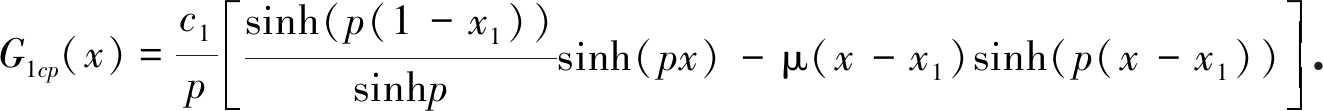(18)

### 2.2 频率方程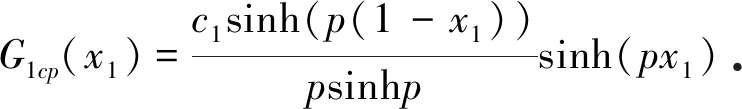(19)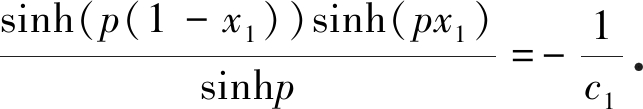(20)

### 2.3 本征向量与和本征函数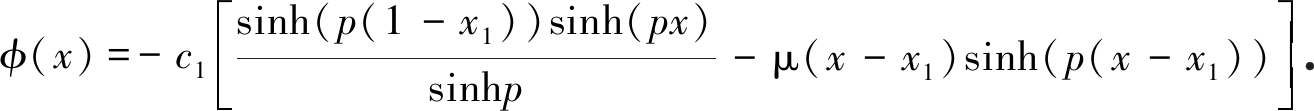(21)

## 3 中点集中阻尼弦本征解的性质

### 3.1 本征值

x1=1/2代入频率方程(20)，该式简化为

tanh(p/2)=-2/c1

(22)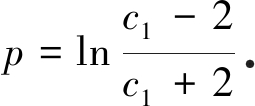(23)

### 3.2 本征函数

x1=1/2代入本征函数式(21)，得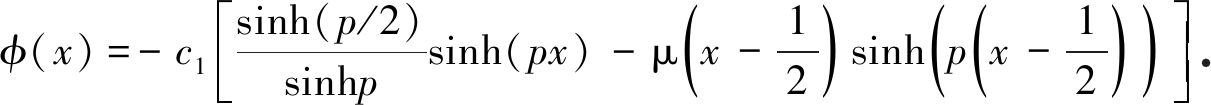(24)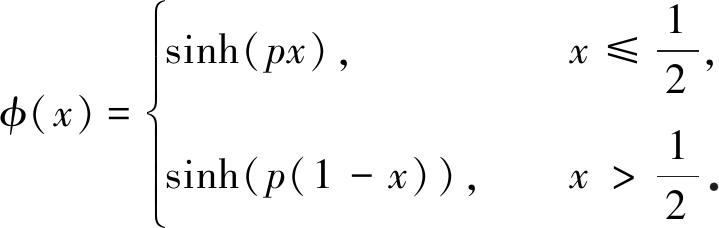(25)

φ(x)=sinh(σx)cos(ωx)+jcosh(σx)sin(ωx)

(26)

### 3.3 本征值与本征函数的性质

3.3.1 大阻尼(c1>2)

pk=ln|(c1-2)/(c1+2)|+j·2sπ， s=0,±1,±2,…

(27)

σ=ln|(c1-2)/(c1+2)|，

(28)

ωk=2sπ， s=0,±1,±2,±3,…

(29)

1) 频率

2) 衰减率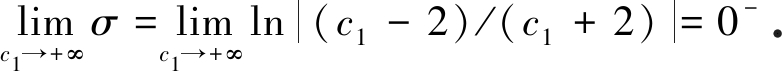(30)

3) 本征函数

φ0(x)=sinh(σx)

(31)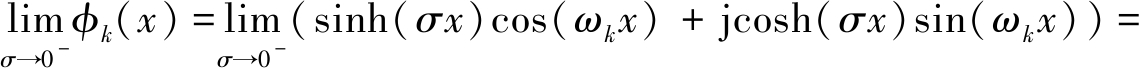σxcos(ωkx)+jsin(ωkx)

(32)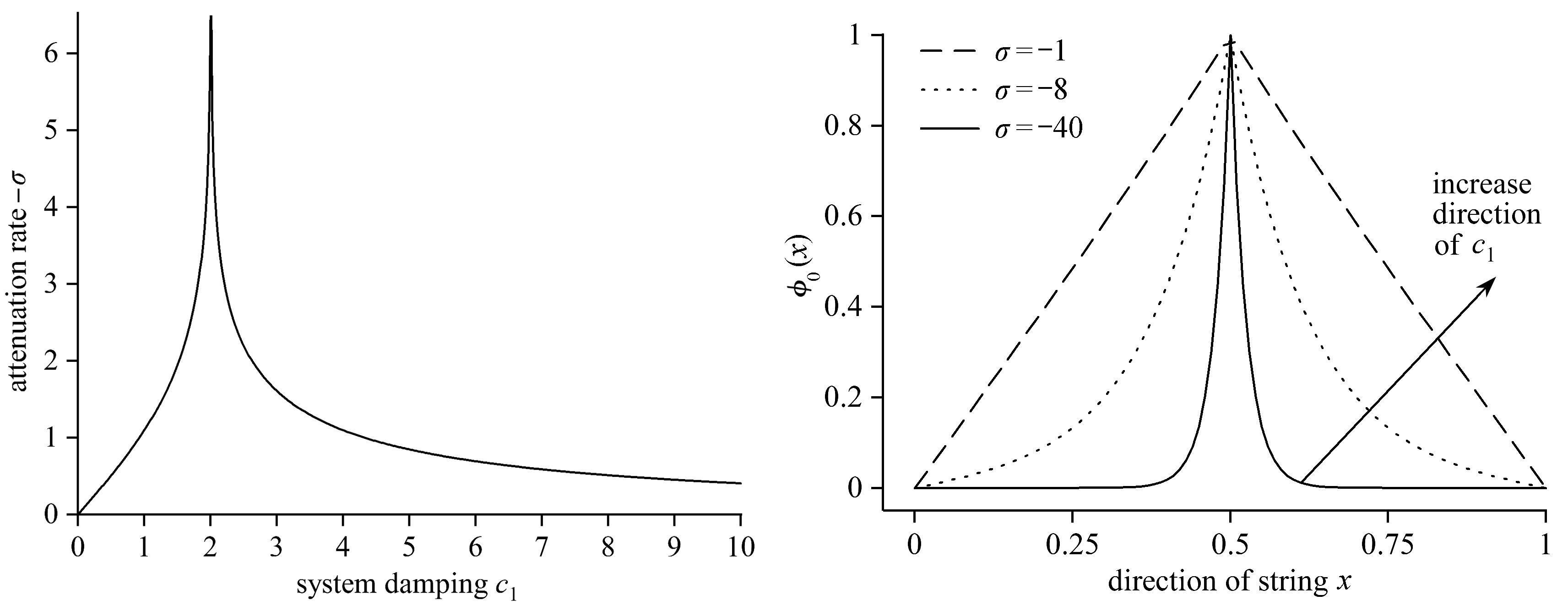Fig. 2 Variation of attenuation rate σ with Fig. 3 The 0th order eigenfunction of the system damping c1 system for c1>2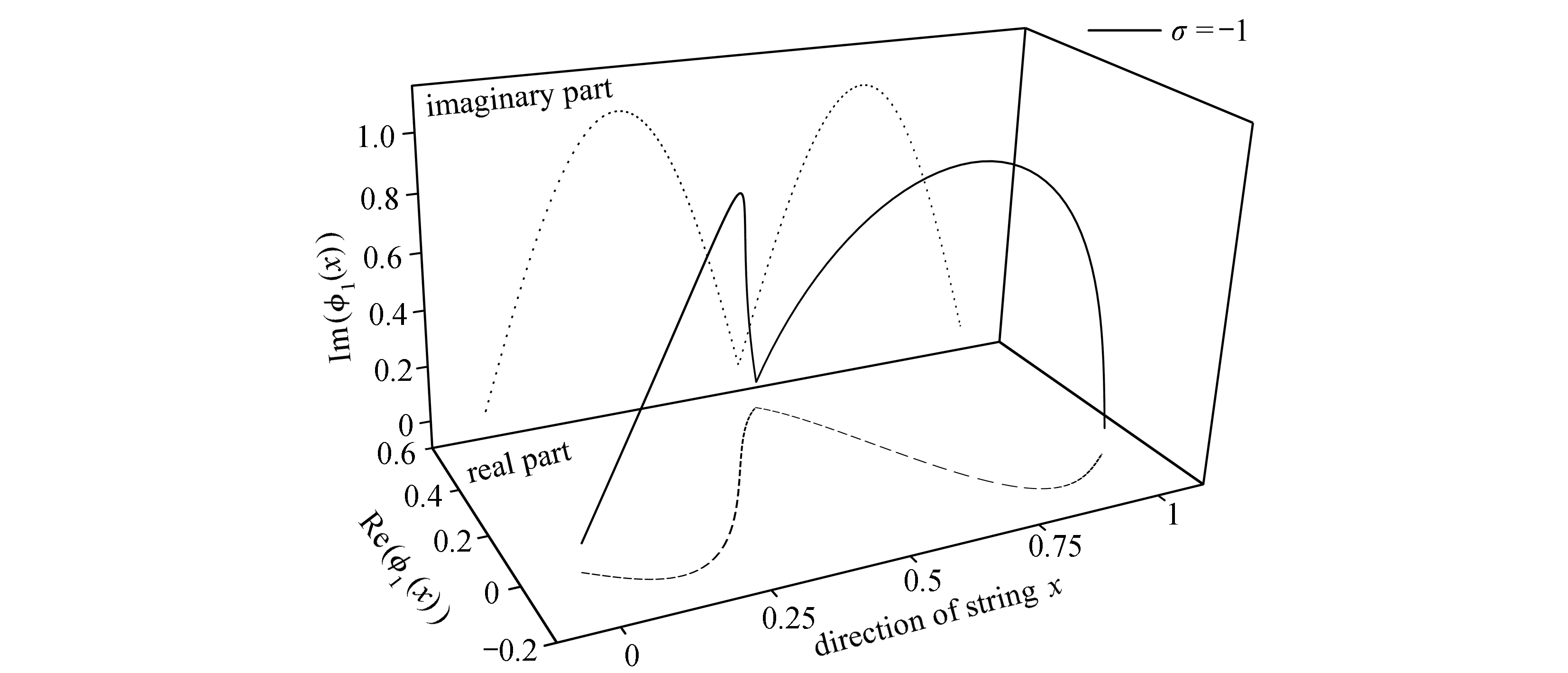(a) 本征函数的空间曲线及其实部、虚部投影
(a) The space curve of the eigenfunction and its real part and imaginary part projections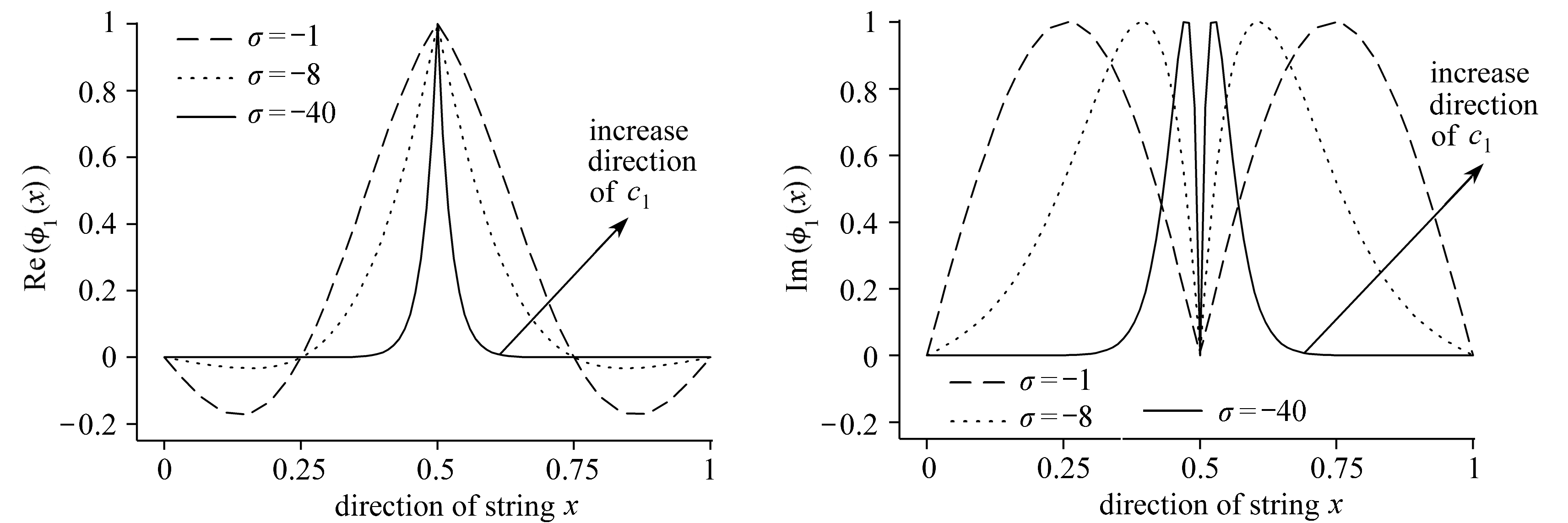(b) 实部形状随c1变化 (c) 虚部形状随c1变化
(b) The real part shape varying with c1 (c) The imaginary part shape varying with c1

Fig. 4 The 1st order eigenfunction of the system for c1>2

3.3.2 适中阻尼(0<c1<2)

pk=ln|(c1-2)/(c1+2)|+j(2s+1)π， s=0,±1,±2,…

(33)

σ=ln|(c1-2)/(c1+2)|，

(34a)

ωk=(2s+1)π, s=0,±1,±2,…

(34b)

1) 频率

2) 衰减率(35)

3) 本征函数σxcos(ωkx)+jsin(ωkx)

(36)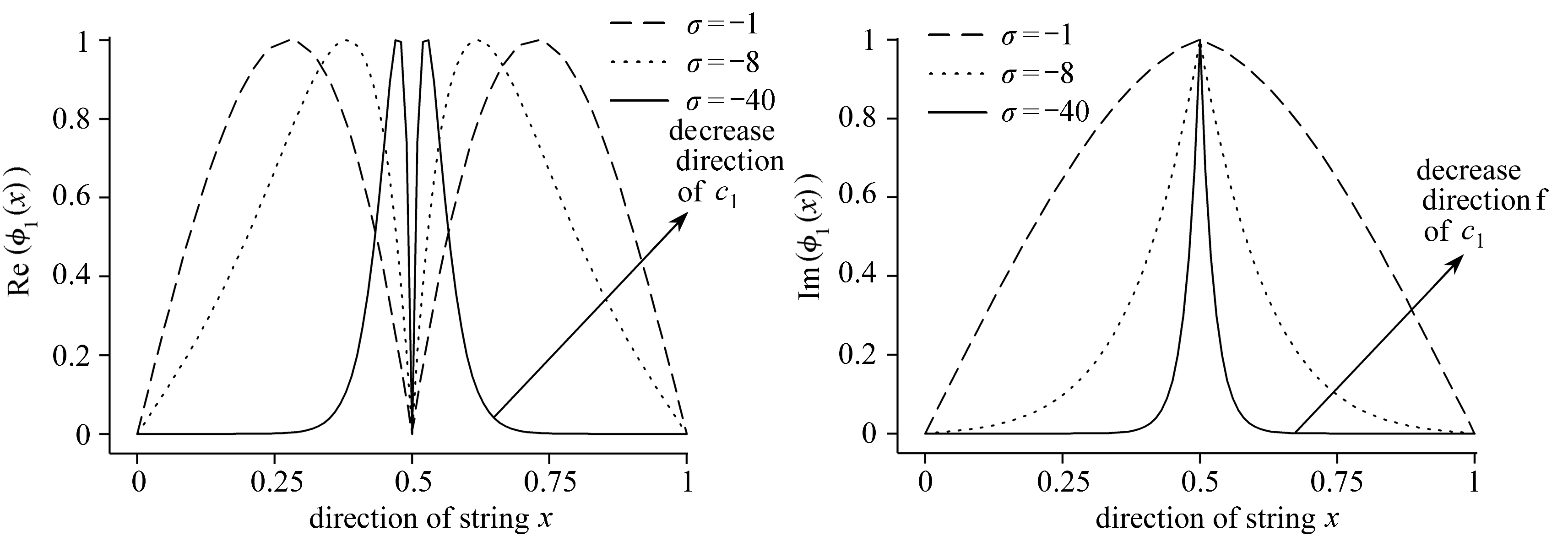(a) 实部形状随c1变化 (b) 虚部形状随c1变化
(a) The real part shape varying with c1 (b) The imaginary part shape varying with c1

Fig. 5 The 1st order eigenfunction of the system for 0<c1<2

3.3.3 特征阻尼(c1=2)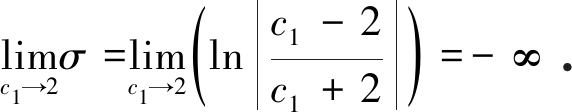(37)

1) 频率

2) 衰减率

3) 本征函数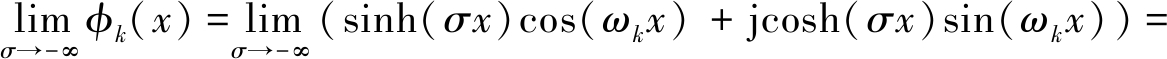e-σx(-cos(ωkx)+jsin(ωkx))/2=

-e-σxe-jωkx/2

(38)

## 4 无衰减自由振动(第二类本征解)

φ(x1)=0，

(39)

-φ″(x)+p2φ(x)=0

(40)

φ(0)=φ(1)=0

(41)

p=jsπ，

(42)

φ(x)=sinh(px)，

(43)

1) 频率

2) 衰减率

3) 本征函数

φv(x)=sin(2sπx)， s=±1,±2,±3,…

(44)

## 5 中点集中阻尼弦自由振动的一般表达式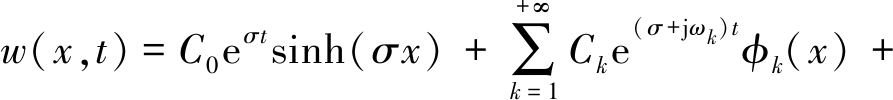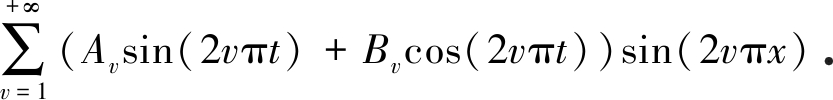(45)

## 6 结果与讨论

1) 集中阻尼弦有三个既区别于连续系统，又区别于离散系统的独特性质：系统频率与阻尼值无关(性质Ⅰ)，阻尼振动的衰减率均相等(性质Ⅱ)，及当阻尼为特定值时，有衰减振动被完全抑制，系统只存在无衰减振动(性质Ⅲ)

2) 当阻尼小于特定值时，系统只有震荡的运动；当阻尼大于特定值时，系统则可同时有震荡运动和非震荡运动

3) 中点集中阻尼弦的无衰减振动均关于中点反对称，有衰减运动(包括0阶的非震荡运动和1阶及以上阶次的震荡运动)均关于中点对称

 CARNE T G. Guy cable design and damping for vertical axis wind turbines: SAND 80-2669[R]. Albuquerque, New Mexico, US: Sandia National Laboratories, 1981.

 YONEDA M, MAEDA K. A study on practical estimation method for structural damping of stay cable with damper[C]//Proceedings of the Canada-Japan Workshop on Bridge Aerodynamics. Ottawa, Canada, 1989.

 PACHECO B M, FUJINO Y, SULEKH A. Estimation curve for modal damping in stay cables with viscous damper[J]. Journal of Structural Engineering, 1993, 119(6): 1961-1979.

 TABATABAI H, MEHRABI A B. Design of mechanical viscous dampers for stay cables[J]. Journal of Bridge Engineering, 2000, 5(2): 114-123.

 YU Z, XU Y L. Mitigation of three-dimensional vibration of inclined sag cable using discrete oil dampers, Ⅰ: formulation[J]. Journal of Sound and Vibration, 1998, 214(4): 659-673.

 MAIN J A, JONES N P. Free vibrations of taut cable with attached damper, Ⅰ: linear viscous damper[J]. Journal of Engineering Mechanics, 2002, 128(10): 1062-1071.

 BATTINI J M. Analysis of dampers for stay cables using nonlinear beam elements[J]. Structures, 2018, 16: 45-49.

 CHEN L, SUN L M, NAGARAJAIAH S. Cable vibration control with both lateral and rotational dampers attached at an intermediate location[J]. Journal of Sound and Vibration, 2016, 377(1): 38-57.

 LAZAR F, NEILD S A, WAGG D J. Vibration suppression of cables using tuned-inerter-dampers[J]. Engineering Structures, 2016, 122(1): 62-71.

# Properties of the Eigen Solution of Taut Strings With Concentrated Damping①

ZHENG Gang, LI Zhangyu, GUO Zengwei, ZHANG Xiaodong

(Co-Constructing State Key Laboratory of Mountain Bridge and Tunnel Engineering by Province and Ministry, Chongqing Jiaotong University, Chongqing 400074, P.R.China)

Abstract: By means of the Dirac δ function, the free-vibration equation of motion for taut strings with concentrated damping, namely the damping hybrid string system, was established and solved. The analytic solution to the eigen problem was obtained for the system with only one single damping dashpot at the midspan, and the properties of the eigen value and the eigen function were analyzed. The dynamic behaviors of the damping hybrid string system, including the frequency-damping relationship, the decay ratio and the full suppression of the motion at the optimal damping, which distinctly differentiate the hybrid system from a continuous system or a discrete system, were identified: 1) the frequency of the hybrid string system is independent of its damping ratio; 2) the decay ratios keep the same for different orders of eigen functions; 3) the decay ratios approach infinity when the damping ratio equals 2, which indicates any damped vibration of the system will be fully suppressed.

Key words: string; concentrated viscous damping; hybrid system; complex mode; non-classic damping

ⓒ 应用数学和力学编委会，ISSN 1000-0887

*收稿日期： 2019-01-08； 修订日期：2019-01-24

DOI: 10.21656/1000-0887.400023

ZHENG Gang, LI Zhangyu, GUO Zengwei, ZHANG Xiaodong. Properties of the eigen solution of taut strings with concentrated damping[J]. Applied Mathematics and Mechanics, 2019, 40(9): 980-990.

Foundation item: The National Natural Science Foundation of China(51978112；51478072；51878106)